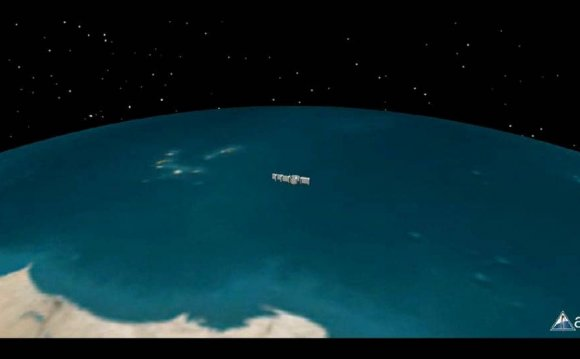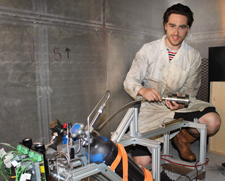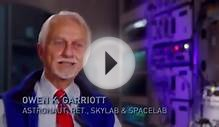# Astronautics and Space Engineering

April 21, 2019Please note that this is an indicative list of modules and is not intended as a definitive list. Those listed here may also be a mixture of core and optional modules.

### Year 1

Introduction to Aerospace and Space Engineering

#### Introduction to Aerospace and Space Engineering

On successful completion of the module, you will be able to:

Describe the characteristics of the atmosphere and explain the basic terminology related to the airflow around a body. Explain the basic theory of flight, flight stability and the operation of aircraft control devices and provide a simple explanation of common engineering design features related to aerodynamic control. Using the general principles of the ATA 100, describe the layout and operation of aircraft major components and systems. Explain the fundamental principles of aircraft gas turbine propulsion and describe the construction and operation of the engines and associated systems. Demonstrate an understanding of the aerospace industry, its regulatory framework and the terminology commonly used. Demonstrate an understanding of the unique demands and benefits of the space environment, the context of space engineering, history of space exploration, and the requirements and features of some example space missions. Thermofluids and Mechanical Principles 1

#### Thermofluids and Mechanical Principles 1

This module introduces the fundamentals of thermofluids (thermodynamics and fluid mechanics) and solid mechanics (statics and dynamics). The thermofluids section covers the key concepts of system, work, heat and the main thermodynamics laws with special reference to their engineering applications. An introduction to main equations of fluid mechanics and dynamics, dimensional analysis, properties of fluids and their measurement methodology and units.

Describe the fundamental properties of a fluid, use correct unit, property tables and charts. State and apply the zeroth, first and second laws of thermodynamics to engineering problems. State the basic equations of fluid mechanics, explain the concepts of pressure, temperature and measurements methods. Describe laminar and turbulent flows and apply continuity, momentum and energy equations to fluid flow. Determine the external and internal forces and moments in simple structures under equilibrium and carry out one-dimensional stress analysis of engineering components in tension, compression and bending modes. Carry out kinematics and kinetics analysis of dynamic systems with constant and variable accelerations. Apply Newton's laws and energy method to engineering components in motion modelled as particles and rigid bodies. Analytical Methods, Computing, Electrical and Electronics Systems

#### Analytical Methods, Computing, Electrical and Electronics Systems

This module introduces the basic concepts from electrical and electronic engineering, using analytical methods. The module embeds a solid foundation in engineering mathematics which is then conceptualised to find solutions to engineering problems. An introduction to basic programming skills applied to engineering problems is also included.

Apply simple principles, laws and theorems to the analysis of electrical and electronic circuits. Describe characteristics of electrical systems, electronic devices and electronic instruments including key concepts such as amplification. Apply basic programming skills to simple engineering problems and demonstrate appreciation of importance of programming in engineering. Perform calculations using matrix algebra, trigonometry and complex numbers. Use calculus to solve engineering problems. Use statistical methods, including probability to an engineering problem. Engineering Design, Materials and Manufacture 1

#### Engineering Design, Materials and Manufacture 1

This module aims to develop competence in the application of the fundamentals of engineering design to a given specification including the manufacture and testing of that design. The module provides an understanding of the structure and synthesis of a broad range of engineering materials, their test methods, structure, implications for manufacture and the control of these structures to produce optimum performance in service. The design part of the module will develop skills in engineering drawings and computer aided design (CAD) and solid modelling together with an introduction to the fundamentals of material science.

Produce and interpret engineering drawings in accordance with international standards. Demonstrate proficiency in the use of solid modelling technologies in the design and development of products. Describe and apply the engineering design process from specification through to design optimisation. Apply a knowledge of molecular structure, crystalline structure and phase transformation to identify different types of materials and to describe their range of properties and applications. Describe the characteristics of a range of common engineering materials including the various failure modes and provide simple analysis using appropriate analytical tools such as the concept of fracture mechanics. Identify and describe common engineering manufacturing processes.
Source: www.kingston.ac.uk
##### RELATED VIDEONOVA SpaceNow 2015: The Future of Space Travel and ...[BBC Space documentary] Travel and the Technology of ...Astrophysics: Documentary on Space, Time, and the Universe ...